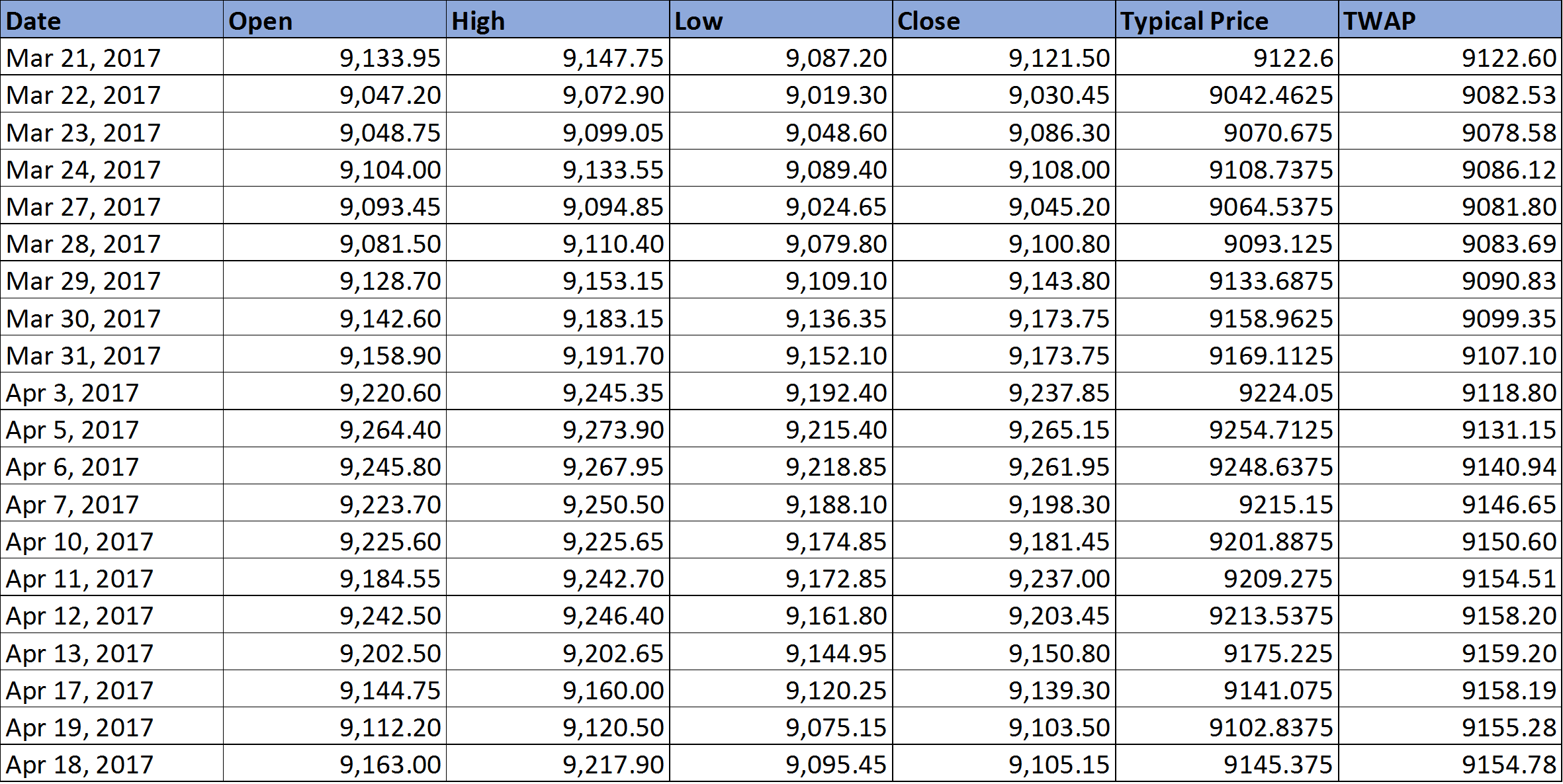Time Weighted Average Price (TWAP) is a variation of the Volume Weighted Average Price (VWAP) primarily utilized by large institutional investors to manage substantial orders without causing market disturbances. TWAP represents the average stock price over a specific time period and sets itself apart from VWAP by excluding volume considerations. In this article, we will demystify the process of calculating TWAP and explore its practical applications in trading, including the availability of a TWAP AFL and an Excel sheet.

### How to Calculate TWAP?

Calculating TWAP is straightforward and relies on finding the average of Open, High, Low, and Close prices for each bar. Here’s a simple example for a 10-period TWAP:

1. Calculate the average of Open, High, Low, and Close values for each of the 10 bars (a1, a2, a3, etc.).
2. Compute the overall average from a1 to a10: TWAP = (a1 + a2 + a3 + … + a10) / 10.

Notably, the calculation of TWAP doesn’t involve complex mathematical equations, making it simpler compared to VWAP. To assist you further, refer to the screenshot below, illustrating the step-by-step process:TWAP Calculation Excel Sheet

### Using TWAP AFL

Below, you’ll find the TWAP AFL code from WiseStockTrader. While initially designed for intraday trading, this AFL can be adapted for daily or weekly timeframes.

```				```
_SECTION_BEGIN("TWAP");
/*
The TWAP for a stock is calculated by Averaging OHLC in each bar then averaging the whole previous bars
Jarrah
*/

Bars_so_far_today = 1 + BarsSince( Day() != Ref(Day(), -1));
StartBar = ValueWhen(TimeNum() == 090000, BarIndex());
TodayClose = Sum(C,Bars_so_far_today);
TodayHigh = Sum(H,Bars_so_far_today);
TodayLow = Sum(L,Bars_so_far_today);
TodayOpen = Sum(O,Bars_so_far_today);
TWAP = (TodayClose + TodayHigh + TodayLow + TodayOpen)/4 ;
IIf (BarIndex() >= StartBar, ATWAP = TWAP / Bars_so_far_today,0);
Plot (ATWAP,"TWAP",colorYellow, styleThick);
_SECTION_END();
```
```

For retail traders who engage in High-Frequency Trading or other quantitative trading strategies, TWAP proves indispensable. It facilitates the astute execution of orders by distributing them uniformly throughout the trading day. Here’s a practical scenario: Suppose your trading algorithm generates a buy signal for 10,000 TCS stocks. To minimize market impact and ensure smooth execution, you should divide this order into smaller portions, say 1,000 shares each, and execute trades based on the TWAP value. Stocks priced below TWAP are generally considered undervalued, while those above TWAP are deemed overvalued. Implementing orders in smaller increments reduces market impact and results in a smoother execution process.

### TWAP vs. VWAP

Let’s compare the fundamental distinctions between TWAP and VWAP:

1. TWAP is solely time-weighted, while VWAP combines time and volume weighting.
2. Small volume trades do not exert influence on TWAP, whereas they can affect VWAP.
3. Calculating TWAP is relatively straightforward, while VWAP calculation is notably complex.
4. With a VWAP trade, around 40% of the transaction occurs in the first half of the trading day, and the remaining 60% takes place in the latter half. In contrast, a TWAP trade typically aims for an evenly distributed 50/50 volume execution during both halves of the day.dexterSeptember 27, 2023dexterSeptember 20, 2023dexterSeptember 20, 2023